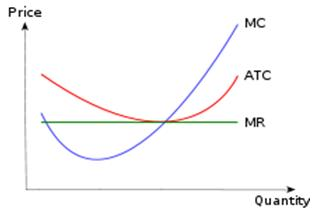# Cost Curve

Posted in Finance, Accounting and Economics Terms, Total Reads: 2938

## Definition: Cost Curve

The cost curve for a company or a product is the graph containing the total cost as a function of the total quantity produced. The graph exhibits the overall profitability of the company or the product by mapping its current position on the graph and comparing it with the best alternative.

This best alternative is the optimum number or quantity of the product or in case of the company, all of its products. This quantity is found by calculating the average total cost, marginal cost of producing the product, the total revenue, average total revenue and the marginal revenue.

The quantity for which the marginal cost curve crosses the marginal revenue curve i.e. marginal cost = marginal revenue, that quantity is the optimum quantity to be produced in case of maximum profit. The total cost curve, average cost curve, marginal cost curve, total revenue curve, average revenue curve and the marginal revenue curve, all incorporate the same axes and hence, they can be shifted from one graph to another, thus, helping in finding the optimum quantity.

Diagram:Hence, this concludes the definition of Cost Curve along with its overview.

Browse the definition and meaning of more terms similar to Cost Curve. The Management Dictionary covers over 7000 business concepts from 6 categories.

Search & Explore : Management Dictionary

Similar Definitions from same Category: